# Digital Control Systems INTRODUCTION Introduction What is a

• Slides: 20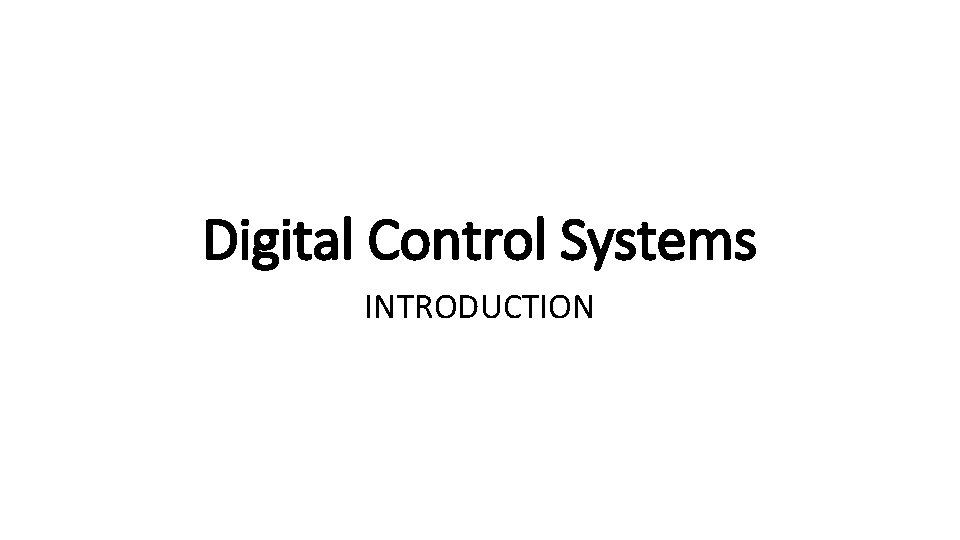Digital Control Systems INTRODUCTION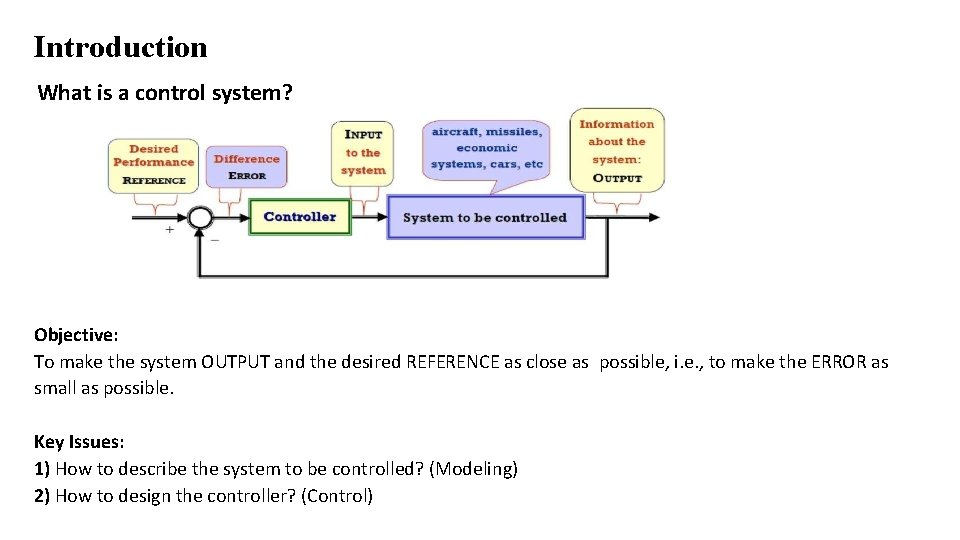Introduction What is a control system? Objective: To make the system OUTPUT and the desired REFERENCE as close as possible, i. e. , to make the ERROR as small as possible. Key Issues: 1) How to describe the system to be controlled? (Modeling) 2) How to design the controller? (Control)Introduction What is important in a control system? Stability • (Transient) response speed • Accuracy Ø dynamic overshooting and oscillation duration Ø Steady state error • Robustness Ø errors in models (uncertainties and nonlinearities) Ø effects of disturbances Ø effects of noises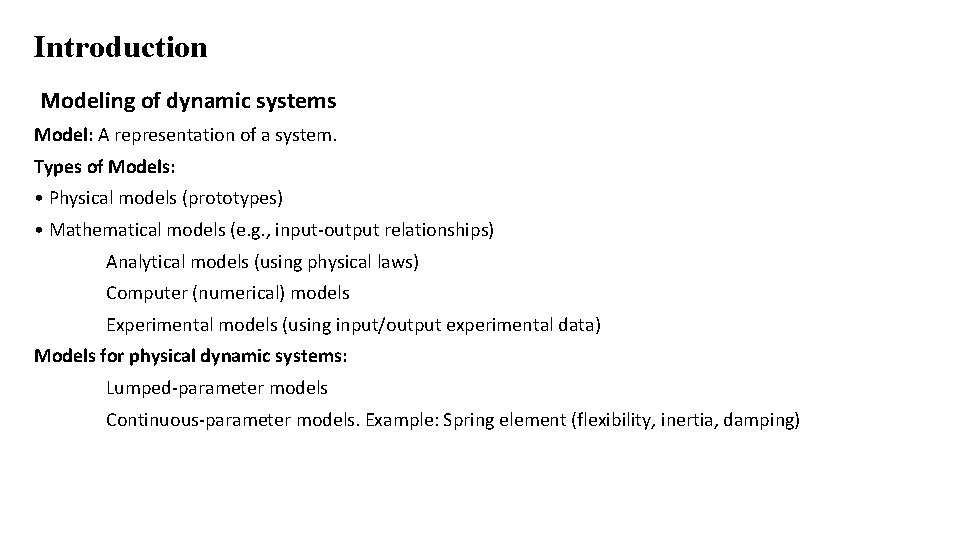Introduction Modeling of dynamic systems Model: A representation of a system. Types of Models: • Physical models (prototypes) • Mathematical models (e. g. , input-output relationships) Analytical models (using physical laws) Computer (numerical) models Experimental models (using input/output experimental data) Models for physical dynamic systems: Lumped-parameter models Continuous-parameter models. Example: Spring element (flexibility, inertia, damping)Introduction Signal categories for identifying control system types Continuous-time signal & quantized signal Continuous-time signal is defined continuously in the time domain. Figure on the left shows a continuoustime signal, represented by x(t). Quantized signal is a signal whose amplitudes are discrete and limited. Figure on the right shows a quantized signal. Analog signal or continuous signal is continuous in time and in amplitude. The real word consists of analog signals.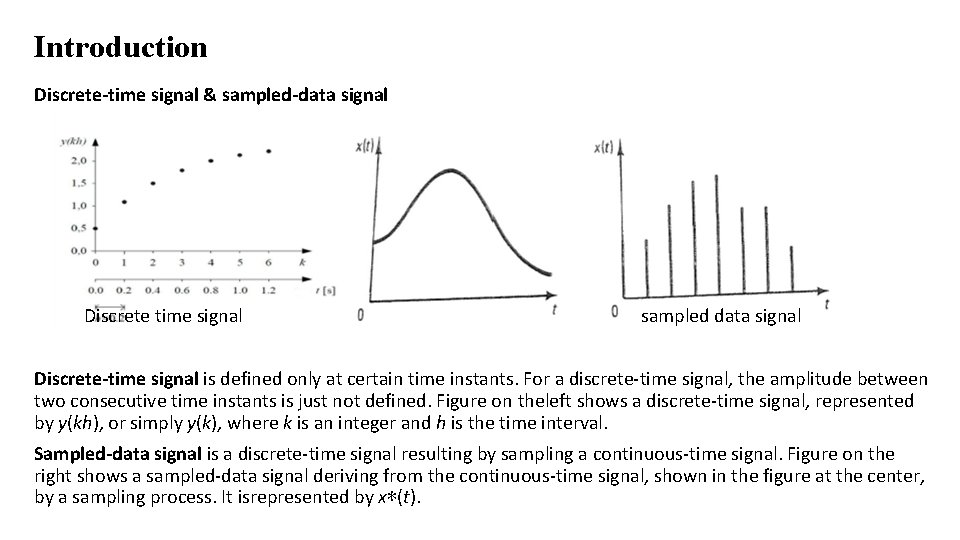Introduction Discrete-time signal & sampled-data signal Discrete time signal sampled data signal Discrete-time signal is defined only at certain time instants. For a discrete-time signal, the amplitude between two consecutive time instants is just not defined. Figure on theleft shows a discrete-time signal, represented by y(kh), or simply y(k), where k is an integer and h is the time interval. Sampled-data signal is a discrete-time signal resulting by sampling a continuous-time signal. Figure on the right shows a sampled-data signal deriving from the continuous-time signal, shown in the figure at the center, by a sampling process. It isrepresented by x∗(t).Introduction Digital signal or binary coded data signal Digital signal is a sequence of binary numbers. In or out from a microprocessor, a semiconductor memory, or a shift register. In practice, a digital signal, as shown in the figures at the bottom, is derived by two processes: sampling and then quantizing.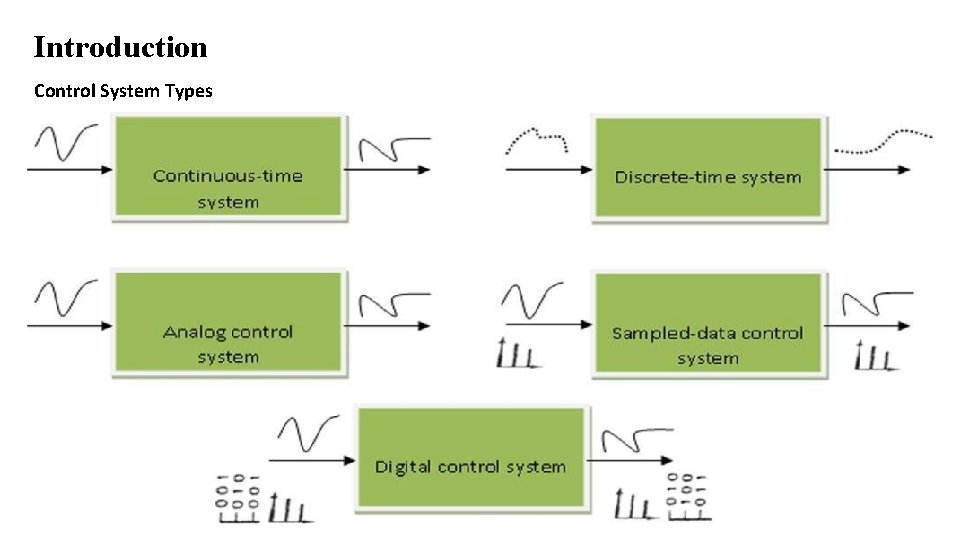Introduction Control System Types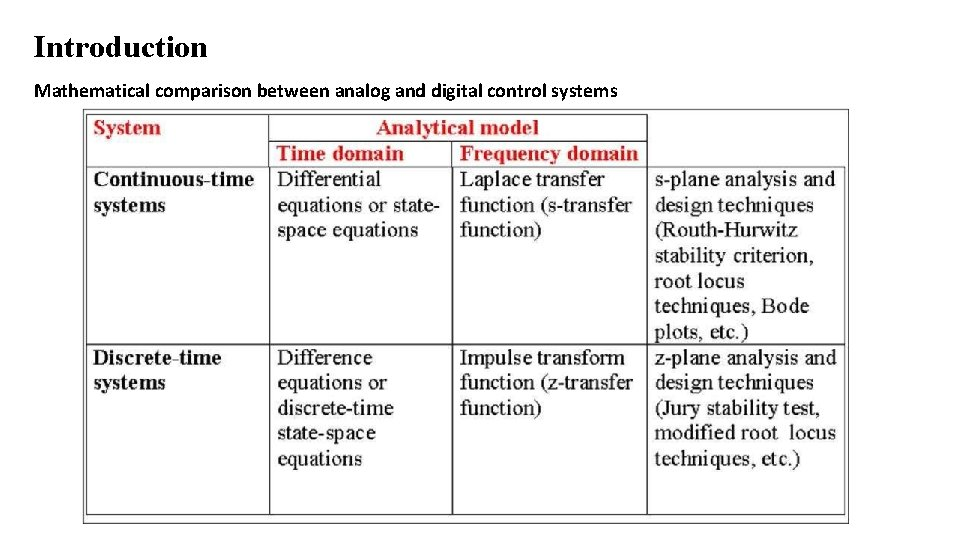Introduction Mathematical comparison between analog and digital control systems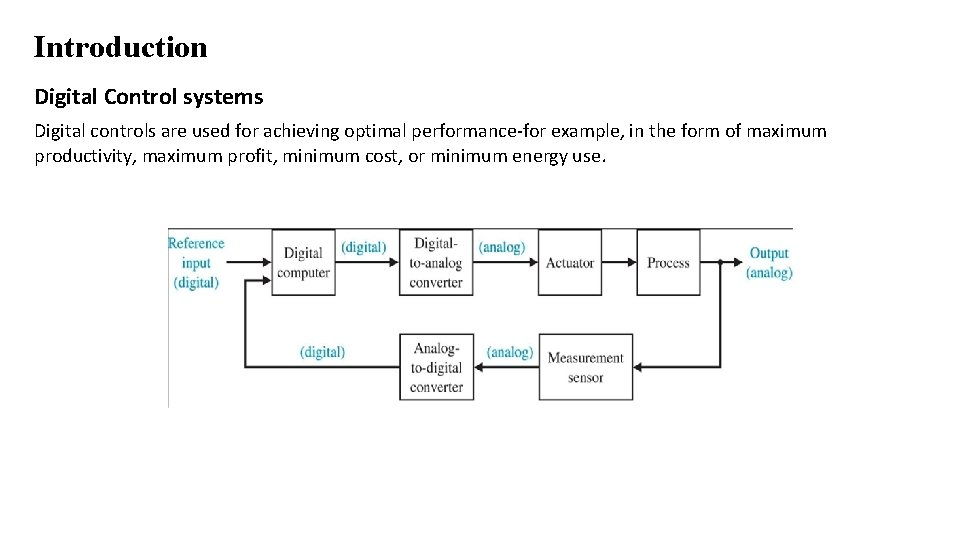Introduction Digital Control systems Digital controls are used for achieving optimal performance-for example, in the form of maximum productivity, maximum profit, minimum cost, or minimum energy use.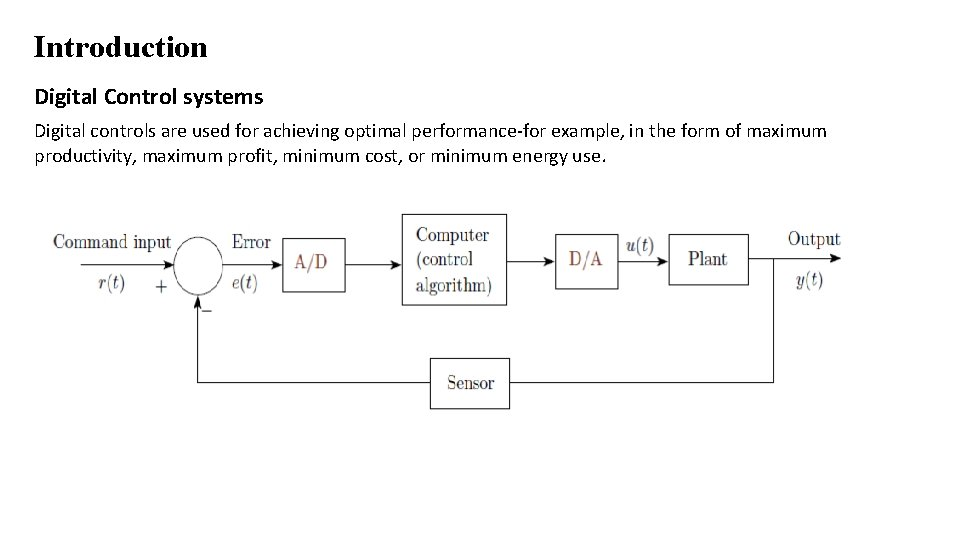Introduction Digital Control systems Digital controls are used for achieving optimal performance-for example, in the form of maximum productivity, maximum profit, minimum cost, or minimum energy use.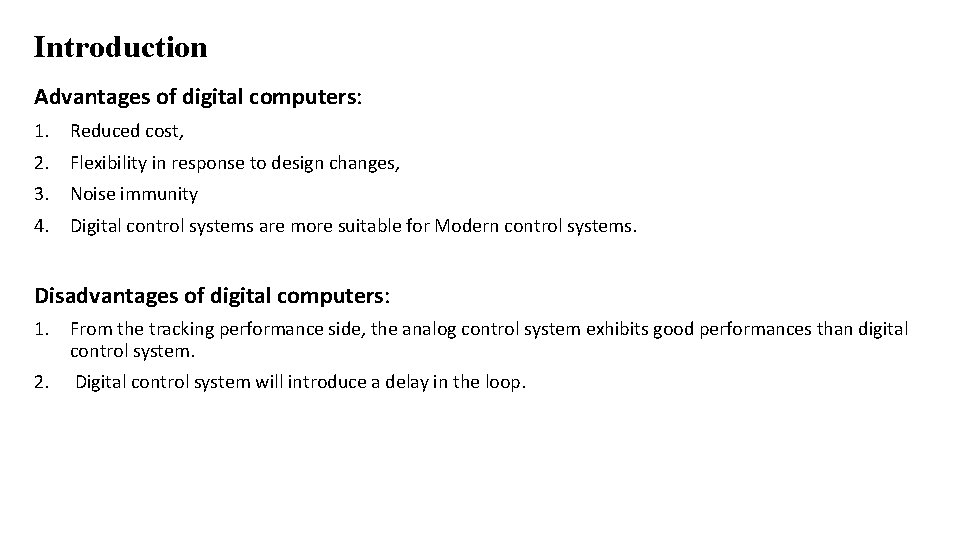Introduction Advantages of digital computers: 1. Reduced cost, 2. Flexibility in response to design changes, 3. Noise immunity 4. Digital control systems are more suitable for Modern control systems. Disadvantages of digital computers: 1. From the tracking performance side, the analog control system exhibits good performances than digital control system. 2. Digital control system will introduce a delay in the loop.Introduction The loop (forward and feedback) contains both analog and digital signals must provide a means for conversion from one form to other to be used by each subsystem. Analog-to-Digital Converter(ADC) A device that converts analog signal to digital signal is called Analog-to-Digital Converter. Digital Analog Converter(DAC) A device that converts digital signals to analog signals is called a Digital Analog Converter.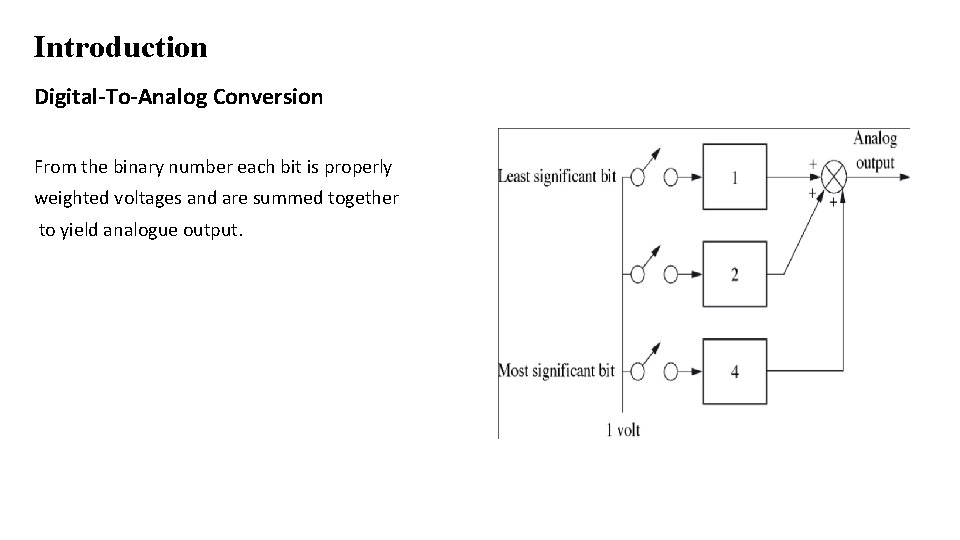Introduction Digital-To-Analog Conversion From the binary number each bit is properly weighted voltages and are summed together to yield analogue output.Introduction Analog-To-Digital Conversion ADC is not instantaneous and needs two-step process. There is a delay between the analog input voltage and the output digital word. In ADC, the analog signal is first converted to a sampled signal and then converted to a sequence of binary numbers, the digital signal.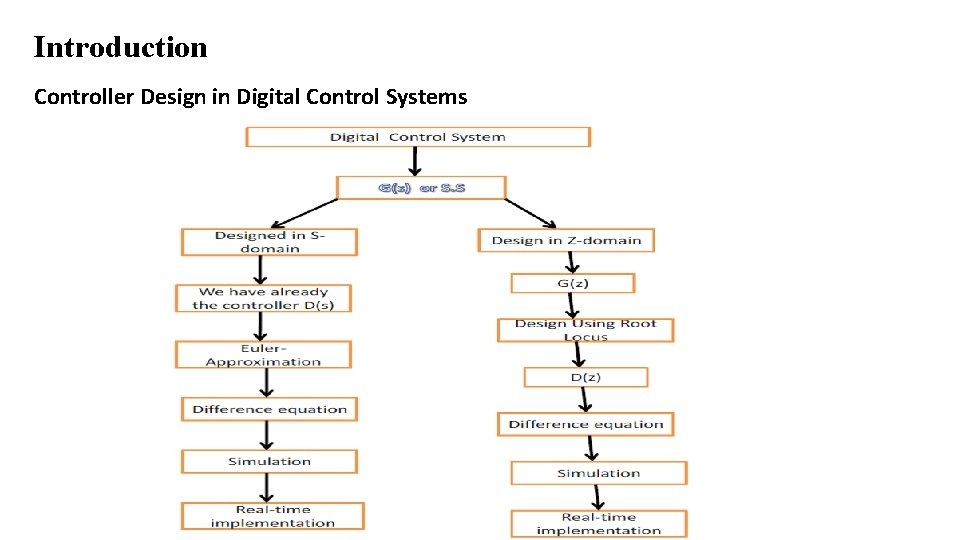Introduction Controller Design in Digital Control SystemsIntroduction Controller design in digital control systems - Design in S-domain Digitization (DIG) or discrete control design The above design works very well if sampling period T is sufficiently small.Introduction How to design a Controller: For the approximation methods, used to convert the continuous controller to digital controller, are: Euler method, Trapezoidal method …etc and we can use forward or backward approximation. forward approximation backward approximation Afterwards, the differential equation of the controller can be transformed to difference equation where this difference equation can be easily programmed as a control algorithm. Note that the sampling time T should be close to zero.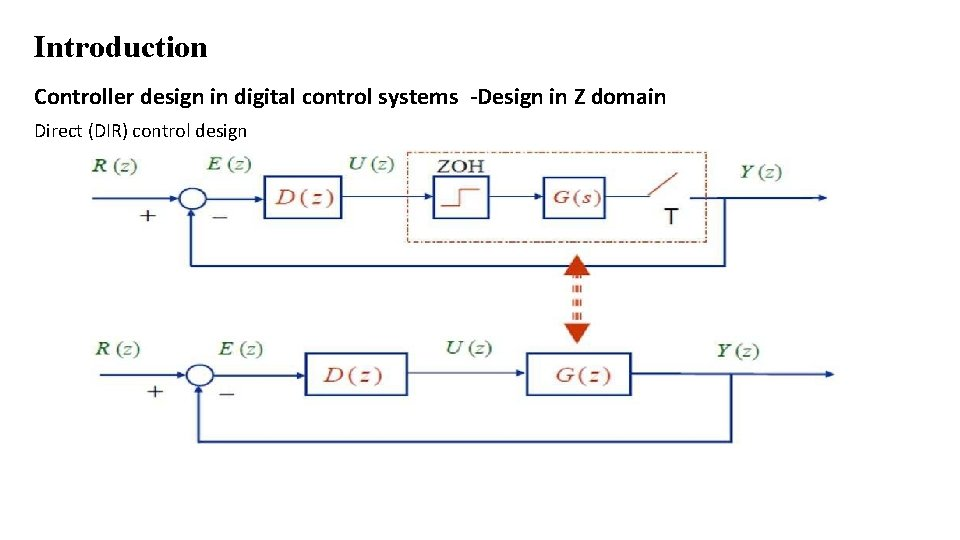Introduction Controller design in digital control systems -Design in Z domain Direct (DIR) control design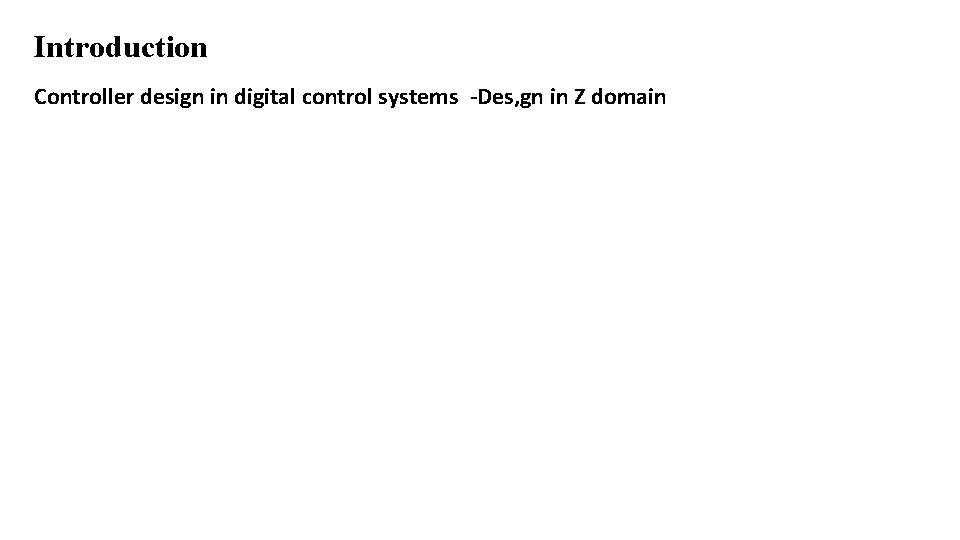Introduction Controller design in digital control systems -Des, gn in Z domain